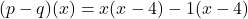Question

￼if p(x)=x*2-1 and q(x)=5(x-1),which expression is equivalent to (p-q)(x)?

1.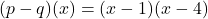Step-by-step explanation:

Given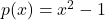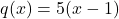Required

(p – q)(x)

This is represented as: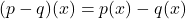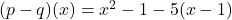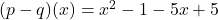Collec like terms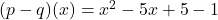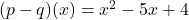Expand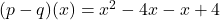Factorize